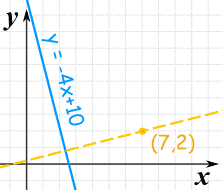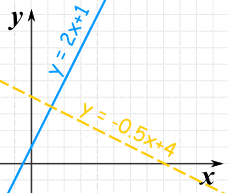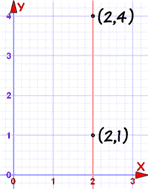# Parallel and Perpendicular Lines

How to use Algebra to find parallel and perpendicular lines.

## Parallel Lines

How do we know when two lines are parallel?

Their slopes are the same!

 The slope is the value m in the equation of a line: y = mx + b### Example:

Find the equation of the line that is:

• parallel to y = 2x + 1
• and passes though the point (5,4)

The slope of y=2x+1 is: 2

The parallel line needs to have the same slope of 2.

We can solve it using the "point-slope" equation of a line:

y − y1 = 2(x − x1)

And then put in the point (5,4):

y − 4 = 2(x − 5)

And that answer is OK, but let's also put it in y = mx + b form:

y − 4 = 2x − 10

y = 2x − 6

### Vertical Lines

But this does not work for vertical lines ... I explain why at the end.

### Not The Same Line

Be careful! They may be the same line (but with a different equation), and so are not parallel.

How do we know if they are really the same line? Check their y-intercepts (where they cross the y-axis) as well as their slope:

### Example: is y = 3x + 2 parallel to y − 2 = 3x ?

For y = 3x + 2: the slope is 3, and y-intercept is 2

For y − 2 = 3x: the slope is 3, and y-intercept is 2

In fact they are the same line and so are not parallel

## Perpendicular Lines

Two lines are Perpendicular when they meet at a right angle (90°).

To find a perpendicular slope:

When one line has a slope of m, a perpendicular line has a slope of −1m

In other words the negative reciprocal### Example:

Find the equation of the line that is

• perpendicular to y = −4x + 10
• and passes though the point (7,2)

The slope of y=−4x+10 is: −4

The negative reciprocal of that slope is:

m = −1−4 = 14

So the perpendicular line will have a slope of 1/4:

y − y1 = (1/4)(x − x1)

And now put in the point (7,2):

y − 2 = (1/4)(x − 7)

And that answer is OK, but let's also put it in "y=mx+b" form:

y − 2 = x/4 − 7/4

y = x/4 + 1/4

## Quick Check of Perpendicular

When we multiply a slope m by its perpendicular slope −1m we get simply −1.

So to quickly check if two lines are perpendicular:

When we multiply their slopes, we get −1

Like this:Are these two lines perpendicular?

 Line Slope y = 2x + 1 2 y = −0.5x + 4 −0.5

When we multiply the two slopes we get:

2 × (−0.5) = −1

Yes, we got −1, so they are perpendicular.

## Vertical Lines

The previous methods work nicely except for a vertical line:In this case the gradient is undefined (as we cannot divide by 0):

m = yA − yBxA − xB = 4 − 12 − 2 = 30 = undefined

So just rely on the fact that:

• a vertical line is parallel to another vertical line.
• a vertical line is perpendicular to a horizontal line (and vice versa).

## Summary

• parallel lines: same slope
• perpendicular lines: negative reciprocal slope (−1/m)